# SAT Math Multiple Choice Question 240: Answer and Explanation

### Test Information

Question: 240

15. A bacteria population, P, can be modeled by the equation P = P010kt, where P0 is the bacteria population at the beginning of the experiment, t is the time in hours since the beginning of the experiment, and k is a positive constant. Which of the following gives the time, t, in terms of k, P, and P0 ?

• A.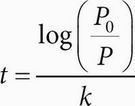• B.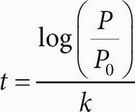• C.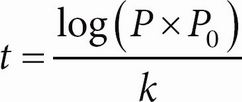• D.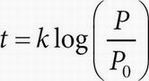BWhenever there are variables in the question and in the answers, think Plugging In. If P0 = 4, k = 2, and t = 3, then P = (4)(10)6 = 4,000,000. Plug these values into the answer choices to see which answer works. Choice (A) becomes 3 =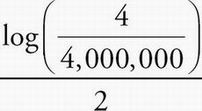. Simplify the right side of the equation to get 3 =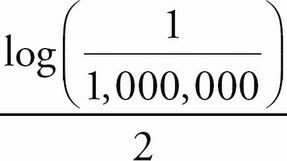, then 3 =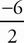, and finally 3 = -3. This isn't true, so eliminate (A). Choice (B) becomes 3 =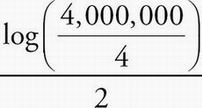. Simplify the right side of the equation to get 3 =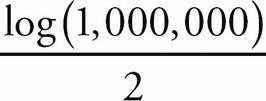, then 3 =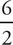, and finally 3 = 3. Keep (B), but check the remaining answer choices just in case. Choice (C) becomes 3 =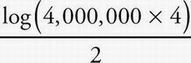. Simplify the right side of the equation to get 3 =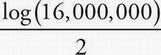, or 3 ≈ 3.6. Eliminate (C). Choice (D) becomes 3 = 2log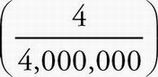. Simplify the right side of the equation to get 3 = 2log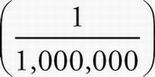, then 3 = 2(-6), and finally 3 = -12. Eliminate (D). The correct answer is (B).